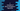# C program to count number of 1 using Brian Kernighan’s Algorithm### C program of Brian Kernighan’s Algorithm to count number of 1 in binary representation of a number :

In this example, we will learn how to find the count of 1 in the binary representation of a number. For example, the binary value of 3 is 0011 and total number of 1 in this representation is 2. Different ways are there to calculate the total count. Brian Kernighan’s Algorithm is one of them. The complexity of this algorithm is O(log(n)).

### How Brian Kernighan’s Algorithm works ?

To understand the algorithm, first take a look into the binary representation from 0 to 10 :

``````0000 = 0
0001 = 1
0010 = 2
0011 = 3
0100 = 4
0101 = 5
0110 = 6
0111 = 7
1000 = 8
1001 = 9
1010 = 10
``````

Let’s try to find how these bits are related : If we subtract 1 from a number , all ‘0’s (if available) to the right of rightmost ‘1’ changes to ‘1’ and the ‘1’ changes to ‘0’. For example, 10 - 1 = 9. 10 is 1010 . Change all 0 of the rightmost 1 to 1 = 1011. Now change the 1 to 0 = 1001 which is the binary representation of 9. Brian Kernighan’s Algorithm is based on this relation. To count the number of bits in a number, first we will subtract 1 from that number and will do bitwise & with itself. What will happen ? The rightmost _1 will become 0 . For example, for 2 and 1, it will be 0010 & 0001. We will do the same process till the final result becomes 0 or 0000. The total number of time required to reach 0 is the count of 1 in that binary number. _

### C program :

``````#include <stdio.h>

int main()
{
//1
int count = 0;
int number;

//2
printf("Enter a number : \n");
scanf("%d", &number);

//3
while (number)
{
//4
number &= (number - 1);
count++;
}

//5
printf("Total number of 1 is = %d \n", count);

return 0;
}``````

### Explanation :

The commented numbers in the above program denotes the steps number below :

1. Create one integer variable count to store the count of 1. Create one more integer variable number to store the value of user input value.
2. Ask the user to enter the number and store it in variable number.
3. Start one while loop. The loop will run till the value of number becomes 0.
4. Do bitwise & of number and number - 1 and set the value to number. Increment the value of count. Do the same process again and again till number becomes 0.
5. After the loop is completed, print the value of count i.e. total number of 1 in binary representation of number.

### Sample Output :

``````Enter a number :
4
Total number of 1 is = 1

Enter a number :
5
Total number of 1 is = 2

Enter a number :
7
Total number of 1 is = 3
``````